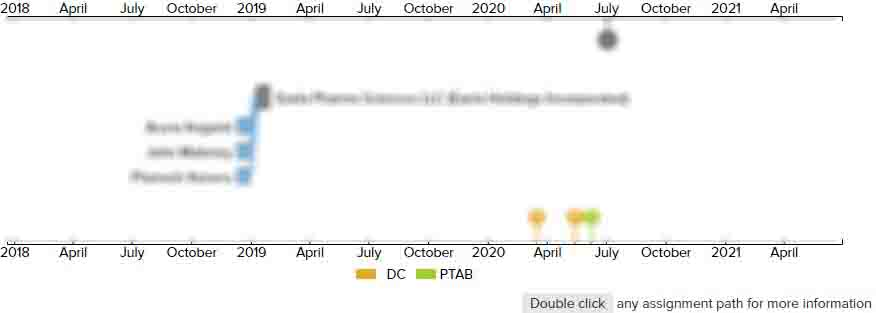# Optical lens for correcting astigmatism

• US 5,016,977 A
• Filed: 02/05/1990
• Issued: 05/21/1991
• Est. Priority Date: 02/06/1989
• Status: Expired due to Fees
##### First Claim
Patent Images

1. A lens for correcting astigmatism, including adjacent hyperbolic- or elliptical-outline diffractive components whose periodicity in two mutually orthogonal X and Y directions intersecting at the axis of the lens and coinciding with the main axes of the hyperbolas or the ellipses are respectively determined by the equations:

•

space="preserve" listing-type="equation">Δ

r.sub.x.sup.2 =2λ

|f.sub.x |; and

space="preserve" listing-type="equation">Δ

r.sub.y.sup.2 =2λ

|f.sub.y |;

in which;

Δ

rx2 represents the periodicity in r2 along the X direction;

Δ

ry2 represents the periodicity in r2 along the Y direction;

λ

represents the means utilization wavelength;

fx represents the desired focal length in the X direction; and

fy represents the desired focal length in the Y direction.

• ##### 1 Assignment
Timeline View
Assignment View×
×
×